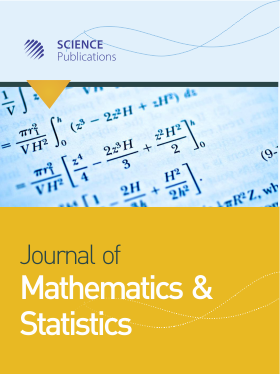Frequency: Continuous
ISSN: 1549-3644 (Print)
ISSN: 1558-6359 (Online)
Research Article Open Access

# Disjoint Sets in Graphs and its Application to Electrical Networks

Ford Lumban Gaol1
• 1 , Afganistan
Journal of Mathematics and Statistics
Volume 7 No. 1, 2011, 73-77

DOI:

Submitted On: 8 February 2011 Published On: 25 March 2011

How to Cite: Gaol, F. L. (2011). Disjoint Sets in Graphs and its Application to Electrical Networks. Journal of Mathematics and Statistics, 7(1), 73-77. https://doi.org/10.3844/jmssp.2011.73.77

## Abstract

Problem statement: One of the well known problems in Telecommunication and Electrical Power System is how to put Electrical Sensor Unit (ESU) in some various selected locations in the system. Approach: This problem was modeled as the vertex covering problems in graphs. The graph modeling of this problem as the minimum vertex covering set problem. Results: The degree covering set of a graph for every vertex is covered by the set minimum cardinality. The minimu of a graph cardinality of a degree covering set of a graph G is the degree covering number γP(G). Conclusion: We show that Degree Covering Set (DCS) problem is NP-complete. In this study, we also give a linear algorithm to solve the DCS for trees. In addition, we investigate theoretical properties of γP (T) in trees T.

• 1,118 Views